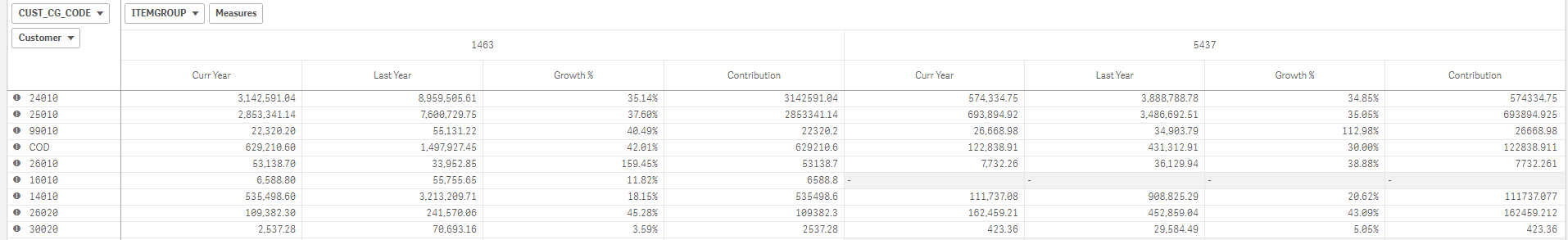New to Qlik Sense

Discussion board where members can get started with Qlik Sense.

Announcements
QlikWorld 2020: Join us May 11 - 14, 2020 in Phoenix, AZ. Register early and save \$400. Learn More
Highlighted
Contributor II

pivot table total

When I am using total function, it is showing total for all columns. how can i find total specific to each column.

Tags (3)
7 RepliesMVP

Re: pivot table total

Could you illustrate by giving us a screenshot or example of what you are looking for?

Contributor II

Re: pivot table totalHere i need to find contribution. So i am using total function. but it gives sum for both columns(1463 & 5437). i need total for rows for each columnMVP

Re: pivot table total

So you need to get the Contribution measure correct - right?

How does the calculation of the contribution look like if it was done on a spreadsheet?

Contributor II

Re: pivot table total

Yes.

Contribution cur year.

Sum(curyear)/ Sum(total <  itemgroup> curyear)MVP

Re: pivot table total

I don't have a clear understanding of your problem.... is it possible to rephrase it? What is wrong and what do you want to get?

Contributor II

Re: pivot table total

sorry for not being clear.

Even if I am using Total qualifier with ITEMGROUP(column), I get same value for both Item group.So to get total for each itemgroup what should be formula.

Contributor II

Re: pivot table total

I can get contribution when i don't have a column.Total<cust_cg_code> gets me total. but when i have column for ITEMGROUP Total<cust_cg_code,ITEMGROUP> IS GIVING SAME VALUE FOR BOTH ITEM GROUP